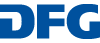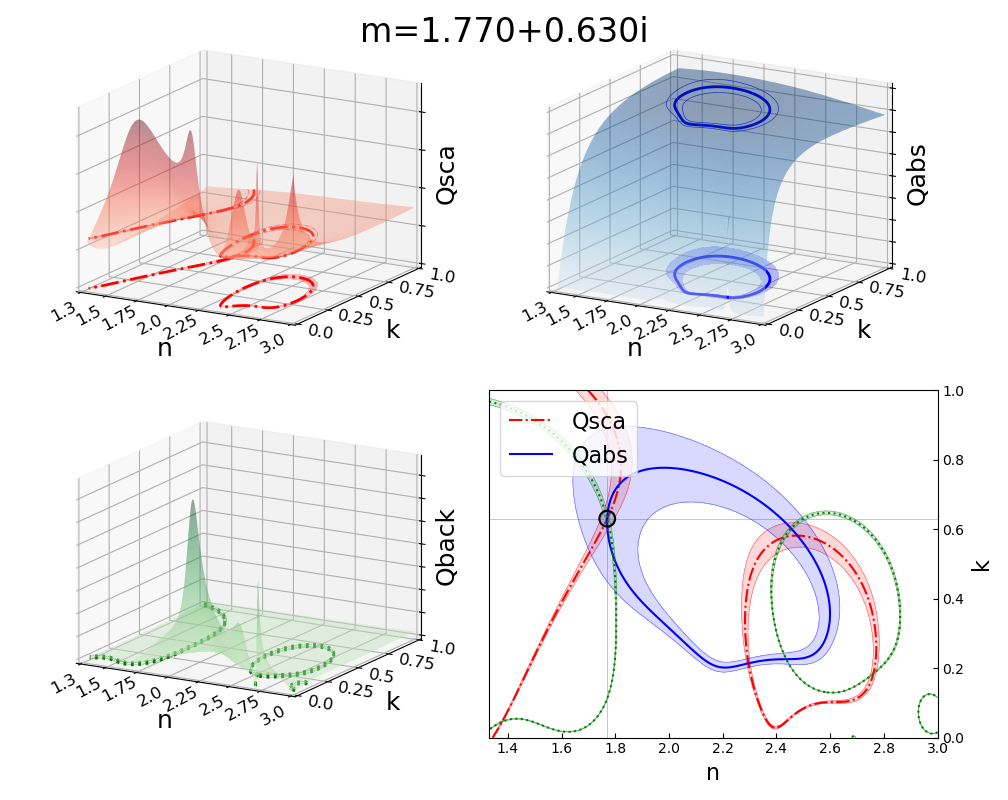You are here:   HomeLight Scattering Software 25 | 10 | 2021
Related / External
Acknowledgement
We acknowledge support of this project by Deutsche Forschungsgemeinschaft (DFG) within the special program "Themenorientierte Informationsnetzwerke".The page is hosted by the University of Bremen.### Nonlocal Conductivity Tensor for a Sphere

Matlab codes for Mie-Type Calculation of the Generalized Electromagnetic Nonlocal Conductivity Tensor for a Sphere and Its Equivalence to the T-Matrix Operator

Mie Simulator GUI

This is a Mie Simulator GUI application. Mie Simulator GUI tool is capable of calculating
- Scattering coefficient
- Scattering cross section
- Reduced scattering coefficient
- Phase function
- S1 and S2
- Average cosine of the phase function
for a single or series of wavelengths### Lorentz-Mie scattering

Lorentz-Mie scattering code in fortran90, based on Hong Du's paper: "Mie-Scattering Calculation, Applied Optics, Vol. 43, Issue 9, pp. 1951-1956 (2004)",

Absphere

Abshere by Kuan Fang Ren is based on the rigorous theory to calculate various physical quantities in the interaction of a light beam with a homogeneous spherical particle or with a concentric layered refractive index gradient. ABSphere allows to calculate: (1) scattering diagrams, (2) radiation pressure (force) and torque exerted by a beam of light on the particle, (3). internal and external electromagnetic fields, (4). extinction, scattering and absorption sections. The forms of the beam considered in ABSphere: (1) circular Gaussian beam. (2) elliptical Gaussian beam. (3) Dungnut beam of 4 different polarizations, (4). Bessel beam (5).

• Link (31 Jul 2015, 23 Sept 2021)### STRATIFY: a comprehensive and versatile MATLAB code for a multilayered sphere

Matlab code by Ilia Rasskazov for electromagnetic properties of a general multilayered (stratified) sphere# MieScatter.jl

Compute Mie scattering in Julia. Mie scattering is the scattering of an electromagnetic plane wave by a homogeneous sphere. Based on a Fortran code by Karri Muinonen.

``````using MieScatter
S, Qsca, Qext, Qback = compute_mie(x, m, N)
S, Qsca, Qext, Qback = compute_mie(x, m, list_of_angles)``````

# Python Mie Scattering package (PyMieScatt)

In addition to over twenty functions for Mie theory calculations, it contains our implementation of a highly visual method for solving the inverse Mie problem for the complex refractive index, given known or assumed size parameter and optical measurements.Mie theory and phase function expansion code by Chris Godsalve.

SMARTIES: Spheroids Modelled Accurately with a Robust Matlab T-matrix Implementation for Electromagnetic ScatteringCode Fortran basé sur la théorie de Lorenz-Mie by Marchant Benjamin`.`